## Refraction

Most of us have a pretty good idea of what reflection is, even before learning about it in physics. We don't have that luck with refraction. Although refraction happens around us all of the time, we're less aware of it.

The best way to think about refraction is like this:

In a vacuum, light moves at, well, the speed of light, which is 3 × 108 m/s. In everything else it slows down. This is because everything else is more "optically dense" than a vacuum. A vacuum is pretty much the absence of anything. Everything else has something in it, and that something slows down light.

When light moves from one object into another it doesn't just pass straight through: it enters the new object, its speed changes, and it's direction or path changes. That means that when light changes speed, it also changes direction. This is what we call refraction.

### Index of Refraction

How much the path of light changes depends on how much it slows down. Or in physics speak, on how much of a difference in optical density there is between the medium it was first traveling in and the object it is entering. Another word for "optical density" is the index of refraction. The index of refraction, or n, is a measure of how much slower light travels in a medium compared to the speed of light in a vacuum.

We calculate the index of refraction using the formula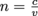where c is the speed of light in a vacuum, or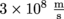, and v is the speed of light in for the object or medium of interest.

Let's practice using the formula to understand it a bit better. So what is the refractive index of a vacuum? Well, the speed of light in a vacuum, or c, is. We're interested in the refractive index of vacuum, so v is also. The refractive index of a vacuum is therefore nvacuum so.The refractive index of a vacuum is therefore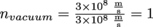.

Now, what about the refractive index of everything else? We already said that light is fastest in a vacuum, so it will never travel faster than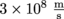.In other words, in the equation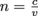,v will always be smaller than c. This means that refractive index of everything other than vacuum is greater than 1. For example, the refractive index of water is 1.3 while the refractive index of diamonds is 2.4.

### Snell's Law

So far we've determined that differences in refractive index between objects change the speed of light and therefore change its path. How much light is refracted depends on the difference in optical density between the two objects. We can calculate the angle of refraction using the law of refraction or Snell's law.

Snell's law is named after Willebrord Snellius. Sounds like something J.K. Rowling would make up.(Source)

Snell's law goes like this: n1sinθ1 = n2sinθ2.

Have we seen this before? Yes, we did in the very first section, but we didn't talk about refraction with Snell's law so much as the index of refraction and how the index determines the bending of light. In plain English, Snell's law says that how much a light ray refracts depends on the refractive indexes of the two materials and on the angle at which light hits a surface, or the incident angle.

Let's look at refraction in a picture since a picture is worth a thousand words:

Now, let's examine the mathematical side of Snell's Law. An imaginary box has a refractive index n2 = 1.5. A light ray (red arrow) travels through water (n1 = 1.3) before it hits this box. Instead of measuring the angle between the light ray and the object, we measure the angle between the light ray and the normal to the object's surface.

The normal is a fancy way of saying a line sticking straight up from the surface at a 90° angle. Take a mop, for instance. (A Swiffer will work, too.) Place the cleaning side of the mop flat on the floor. Now hold it so that the handle points straight up at the ceiling. The handle is what we would call normal (perpendicular) to the floor.

One of the easiest ways to sink our midterm ship is to forget about the normal. Here's a reminder: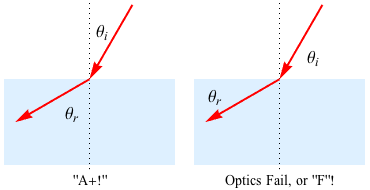Back to our example. Let's fill in some numbers: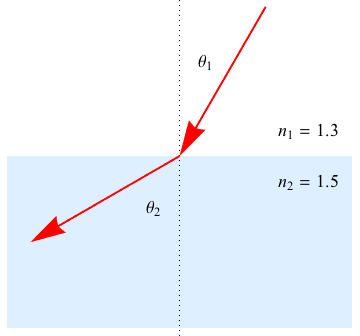Now based on Snell's law, without knowing anything else, we can determine whether the light gets bent towards or away from the normal, just by comparing the two indices of refraction in our equation, n1sinθ1 = n2sinθ2.

In our case n2 is 1.5 and n1 is 1.3, so for light to obey Snell's law (and who would disobey Willebrord?) θ2 has to be smaller than θ1. A smaller θ2 means that the light beam gets refracted or bent towards the normal.

Time to memorize:

If n1 > n2, light will bend away from the normal.
If n1 < n2, light will bend towards the normal.

Actually, as long as we memorize Snell's law in mathematical form, we can figure out the rest. Another brain cell saved to memorize another day.

Mathematics is all fine and dandy, but how about a real-life occurrence?

Actually, we have been duped by indices of refractions before. We've all dropped something into a body of water before, a pair of swim goggles, a set of keys, a cell phone (that one hurt). We misjudge the depth of the objects underwater because of the index of refraction. One-hundred percent of the time the keys and the bottom of the pool are much deeper than they appear to be because nwater > nair, that means that light beams coming from the keys are bent away from the normal as they move from water to air. To our eyes, this bending makes it look as if the light beam came from somewhere much closer to the water surface.

Now that we have a better grasp of indices of refraction, let's revisit total internal reflection again. Here's a picture: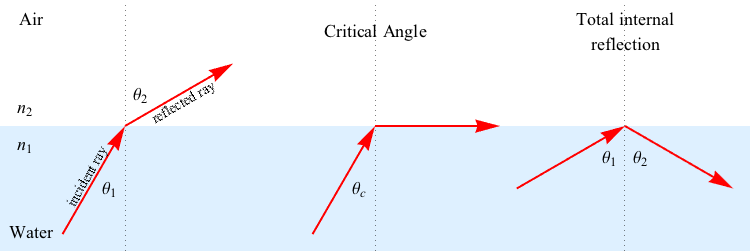(Source)

A light ray passing through a medium with a relatively high angle would bend a larger amount in entering a material with a lower index of refraction, such as air. Once the refraction requires an angle greater than 90˚, BOOM, we have total internal reflection on our hands. Right at 90˚, though, the light travels along the interface between the two. This sweet spot of refraction is called the critical angle.

Let's play. Let's find the critical angle between air and glass.

The index of refraction for air is n2 = 1, and for glass is n1 = 1.5. That's not enough information, though. We need an angle, and whaddaya know, we have one: θ2 = 90˚. Now, we can use Snell's Law to solve for θ1 = 42˚ in 1.5 sin θ1 = 1 sin 90.

The critical angle for a medium depends on both refractive indices, so it changes with the exchange of either material for another. The existence of a critical angle also requires that n1 > n2.

### Common Mistakes

It's easy to forget that the angle is defined with respect to the normal and not the surface of the material, but, no matter what, don't do it. If you do, the math breaks, and if the math breaks…oh, the horror…

### Brain Snack

Refraction appears in literature too. Think of any invisible character, such as the Invisible Man, and you can bet your bottom dollar that the author had optics on her mind (probably). Overthink about any of these fictional works by trying to understand their characters' invisibility not only as symbolic but as real given what you now know about optics. Refraction is behind all invisibility, after all.